## a very occasional diary.nondescript

## 2010-02-04

### The Hunt for Addi(c)tive Monster 2.

In the previous post, we were looking for a monster—a nonlinear additive function. We found that such a function is extremely pathological: it is nowhere locally monotone, nowhere continuous and nowhere locally bounded. Worse than that, it's easy to prove that the graph of a monster is dense in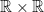, that is, for every x and y, an arbitrary neighborhood of x contains a point that monster sends arbitrarily close to y.

Recall our attempt to construct a monster. Any additive function is linear on any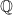-set and is fully determined on this set by a value it has in any single of its points. Our Direchlet function derived monster failed (or rather fell) because the slopes an additive function has on different-sets are not independent. Indeed, given that f has a slope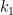on a-set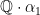and a slope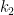on a-set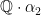, it has to have a slope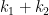on a-set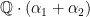. This shows a way to construct a monster: one has to find a collection B of real numbers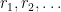such that (i) every real number can be represented as a sum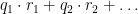, with rational coefficients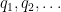of which only finite number is non-zero (so that the sum is defined) and (ii) that such representation is unique. Then one can select arbitrary values on elements of B and take moster's value onto be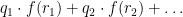, which is well-defined thanks to (ii).

Looks familiar? It should be: the definition of B is exactly the definition of a basis of a vector space. Real numbers can be added to each other and multiplied by rationals and, therefore, form a vector space over. This space is very different from a usual one-dimensional vector space real numbers form over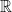(i.e., over themselves).

After a streak of bad and unlikely properties that a monster has, we now got something positive: a monster exists if and only ifas a vector space overhas a basis. Does it?

But of course. Any vector space has a basis—this is a general theorem almost immediately following from the Zorn's lemma. The basis we are looking for even got a name of its own: Hamel basis.

At last we stumbled across the whole family on monsters. Specifically, there exists a set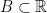and a function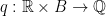such that every real number r can be uniquely represented as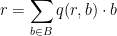where only finite number of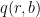are non-zero for a given r. From this it immediately follows that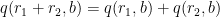.

Take an arbitrary function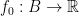, and define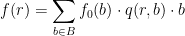Now,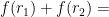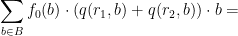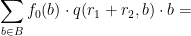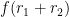that is, f is additive. Intuitively,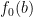is a slope f has at the-set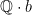. f is linear if and only if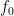is a constant function, in all other cases f is a monster. If one takes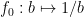, then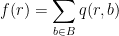is an especially weird monster function: it takes only rational values!

Note that almost all additive functions are, after all, monsters—only very small sub-set of them is linear.

1.2.